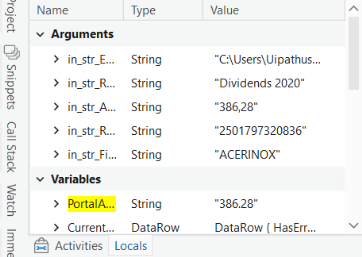# Compare Numeric Values

Look at output panel
386.29<>386.28 Two values not matching but the difference is only 0.01, so how can i ignore this small difference while checking that value is matching or not?
I put three conditions to validate the record other two conditions satisfies but this amount matching condition is not satisfies due to this small difference

Hi

Have you tried converting to ‘Int32’ which will be a whole number (no decimals) so it will be 386. Then you can compare the two numbers?

Create a new variable called:
int_PortalA (Int32)

Convert the two values to Int32 using an Assign:
int_PortalA = CInt(PortalA)

Then simply compare with an ‘IF’ activity.Hopefully this helps

Cheers

Steve

Try like @Steven_McKeering suggestion and you can also try to round the both the values and compare

Regards
Sudharsan

1 Like

Math.Round(Convert.ToDouble(CurrentRow(“Claimed tax”).ToString.Trim.Replace(“,”, “.”)), 2) =Math.Round(Convert.ToDouble(in_str_AmountFromPortal.Trim.Replace(“.”, “”).Replace(“,”, “.”)), 2)

i’m using this condition

Okay Try like this @pravin_bindage

``````Math.Ceiling(Convert.ToDouble(CurrentRow(“Claimed tax”).ToString.Trim.Replace(“,”, “.”)), 2) =Math.Ceiling(Convert.ToDouble(in_str_AmountFromPortal.Trim.Replace(“.”, “”).Replace(“,”, “.”)), 2)
``````

Regards
Sudharsan

2 Likes

this error shows when i use Math.Ceiling insted of Math.Round @Sudharsan_Ka

@pravin_bindage

Remove that “,2” from both the sides and try once

Regards
Sudharsan

1 Like

This topic was automatically closed 3 days after the last reply. New replies are no longer allowed.